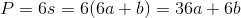# SSAT Upper Level Math : How to find the perimeter of a hexagon

## Example Questions

### Example Question #1 : Areas And Perimeters Of Polygons

A regular hexagon has perimeter 9 meters. Give the length of one side in millimeters.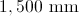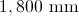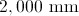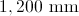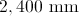Explanation:

One meter is equal to 1,000 millimeters, so the perimeter of 9 meters can be expressed as:

9 meters =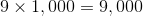millimeters.

Since the six sides of a regular hexagon are congruent, divide by 6: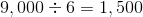millimeters.

### Example Question #2 : How To Find The Perimeter Of A Hexagon

A hexagon with perimeter 60 has four congruent sides of length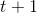. Its other two sides are congruent to each other. Give the length of each of those other sides in terms of.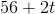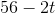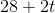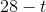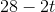Explanation:

The perimeter of a polygon is the sum of the lengths of its sides. Let: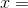Length of one of those other two sides

Now we can set up an equation and solve it forin terms of: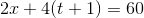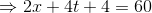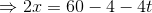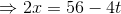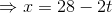### Example Question #3 : How To Find The Perimeter Of A Hexagon

Two sides of a hexagon have a length of, two other sides have the length of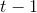, and the rest of the sides have the length of. Give the perimeter of the hexagon.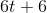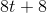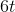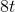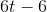Explanation:

The perimeter of a polygon is the sum of the lengths of its sides. So we can write: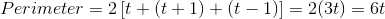### Example Question #4 : How To Find The Perimeter Of A Hexagon

A regular hexagon has perimeter 15 feet. Give the length of one side in inches.Explanation:

As the six sides of a regular hexagon are congruent, we can write: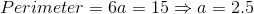feet;is the length of each side.

One feet is equal to 12 inches, so we can write: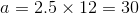inches

### Example Question #1 : Areas And Perimeters Of Polygons

Each interior angle of a hexagon is 120 degrees and the perimeter of the hexagon is 120 inches. Find the length of each side of the hexagon.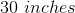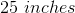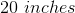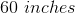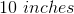Explanation:

Since each interior angle of a hexagon is 120 degrees, we have a regular hexagon with identical side lengths. And we know that the perimeter of a polygon is the sum of the lengths of its sides. So we can write: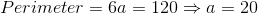inches

### Example Question #6 : How To Find The Perimeter Of A Hexagon

A hexagon with perimeter of 48 has three congruent sides of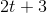. Its other three sides are congruent to each other with the length of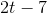. Find.Explanation:

The perimeter of a polygon is the sum of the lengths of its sides. Since three sides are congruent with the length ofand the rest of the sides have the length ofwe can write: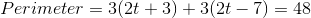Now we should solve the equation for: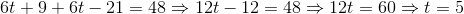### Example Question #7 : How To Find The Perimeter Of A Hexagon

A regular pentagon has sidelength one foot; a regular hexagon has sidelength ten inches. The perimeter of a regular octagon is the sum of the perimeters of the pentagon and the hexagon. What is the measure of one side of the octagon?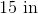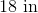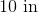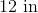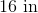Explanation:

A regular polygon has all of its sides the same length. The pentagon has perimeter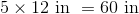; the hexagon has perimeter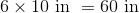. The sum of the perimeters is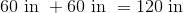, which is the perimeter of the octagon; each side of the octagon has length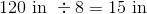.

### Example Question #8 : How To Find The Perimeter Of A Hexagon

Find the perimeter of a hexagon with a side length of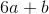.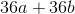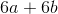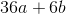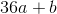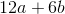Explanation:

A hexagon has six sides.  The perimeter of a hexagon is: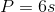Substitute the side length.